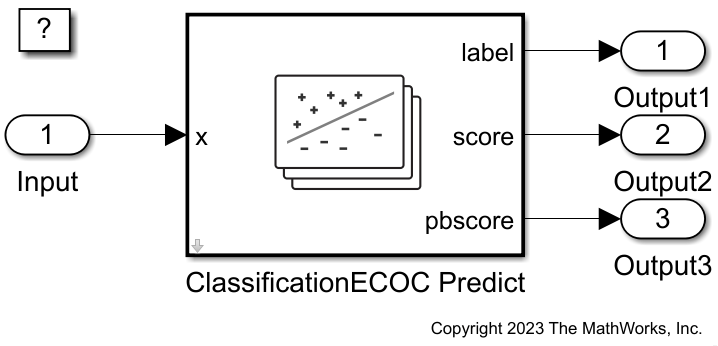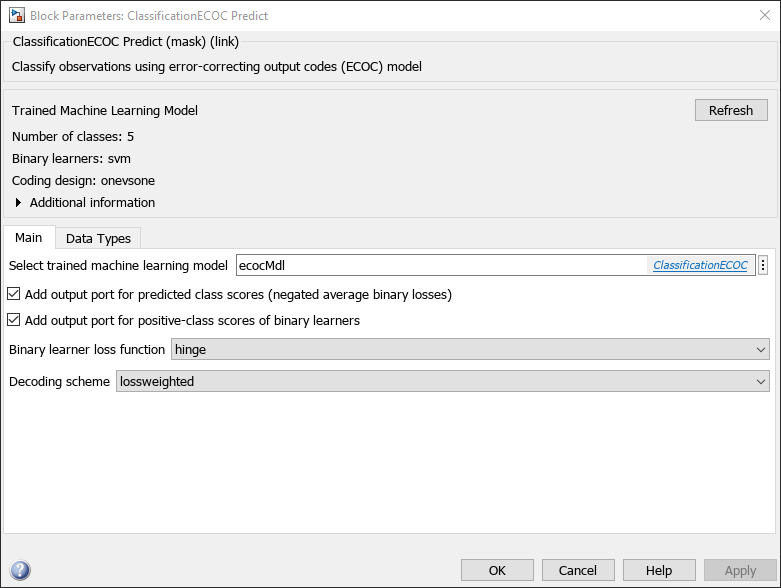# Predict Class Labels Using ClassificationECOC Predict Block

Since R2023a

This example shows how to use the ClassificationECOC Predict block for label prediction in Simulink®. The block accepts an observation (predictor data) and returns the predicted class label, class scores for the observation, and positive-class scores of binary learners, using the trained error-correcting output codes (ECOC) classification model.

### Train Classification Model

Load the `humanactivity` data set. This data set contains 24,075 observations of five physical human activities: Sitting, Standing, Walking, Running, and Dancing. Each observation has 60 features extracted from acceleration data measured by smartphone accelerometer sensors.

`load humanactivity`

Create the predictor `X` as a numeric matrix that contains 60 features for 24,075 observations. Create the class labels `Y` as a numeric vector that contains the activity IDs in integers: 1, 2, 3, 4, and 5 representing Sitting, Standing, Walking, Running, and Dancing, respectively.

```X = feat; Y = actid;```

Randomly partition observations into a training set and a test set with stratification, using the class information in `Y`. Use approximately 80% of the observations to train an ECOC model, and 20% of the observations to test the performance of the trained model on new data.

```rng("default") % For reproducibility of the partition cv = cvpartition(Y,"Holdout",0.20);```

Extract the training and test indices.

```trainingInds = training(cv); testInds = test(cv);```

Specify the training and test data sets.

```XTrain = X(trainingInds,:); YTrain = Y(trainingInds); XTest = X(testInds,:); YTest = Y(testInds);```

Train an ECOC classification model by passing the training data `XTrain` and `YTrain` to the `fitcecoc` function.

`ecocMdl = fitcecoc(XTrain,YTrain);`

`ecocMdl` is a `ClassificationECOC` model. You can use dot notation to access the properties of `ecocMdl`. For example, enter `ecocMdl.CodingMatrix` to display the coding design matrix of the model.

`ecocMdl.CodingMatrix`
```ans = 5×10 1 1 1 1 0 0 0 0 0 0 -1 0 0 0 1 1 1 0 0 0 0 -1 0 0 -1 0 0 1 1 0 0 0 -1 0 0 -1 0 -1 0 1 0 0 0 -1 0 0 -1 0 -1 -1 ```

Each row of the coding design matrix corresponds to a class, and each column corresponds to a binary learner. For example, the first binary learner is for classes 1 and 2, and the fourth binary learner is for classes 1 and 5, where both learners assume class 1 as a positive class.

Enter `ecocMdl.BinaryLearners` to display the model type of each binary learner.

`ecocMdl.BinaryLearners`
```ans=10×1 cell array {1x1 classreg.learning.classif.CompactClassificationSVM} {1x1 classreg.learning.classif.CompactClassificationSVM} {1x1 classreg.learning.classif.CompactClassificationSVM} {1x1 classreg.learning.classif.CompactClassificationSVM} {1x1 classreg.learning.classif.CompactClassificationSVM} {1x1 classreg.learning.classif.CompactClassificationSVM} {1x1 classreg.learning.classif.CompactClassificationSVM} {1x1 classreg.learning.classif.CompactClassificationSVM} {1x1 classreg.learning.classif.CompactClassificationSVM} {1x1 classreg.learning.classif.CompactClassificationSVM} ```

Enter `ecocMdl.BinaryLoss` to display the binary learner loss function.

`ecocMdl.BinaryLoss`
```ans = 'hinge' ```

To classify a new observation, the model computes positive-class classification scores for the binary learners, applies the hinge function to the scores to compute the binary losses, and then combines the binary losses into the classification scores using the loss-weighted decoding scheme.

Compute and display the label, classification scores, and positive-class scores for a new observation by using the `predict` function.

```[label,scores,pbscores] = predict(ecocMdl,XTest(1,:)); label```
```label = 1 ```
`scores`
```scores = 1×5 0 -0.7281 -1.1774 -2.4186 -2.1109 ```
`pbscores'`
```ans = 10×1 4.8247 2.6695 2.4870 1.9088 4.7496 7.5894 9.4470 3.8461 2.5316 -1.4261 ```

The ClassificationECOC Predict block can return the three outputs for each observation you pass to the block.

This example provides the Simulink model `slexClassificationECOCPredictExample.slx`, which includes the ClassificationECOC Predict block. You can open the Simulink model or create a new model as described in this section.

Open the Simulink model `slexClassificationECOCPredictExample.slx`.

```SimMdlName = "slexClassificationECOCPredictExample"; open_system(SimMdlName)```If you open the Simulink model, then the software runs the code in the `PreLoadFcn` callback function before loading the Simulink model. The `PreLoadFcn` callback function of `slexClassificationECOCPredictExample` includes code to check if your workspace contains the `ecocMdl` variable for the trained model. If the workspace does not contain the variable, `PreLoadFcn` loads the sample data, trains the ECOC model, and creates an input signal for the Simulink model. To view the callback function, in the Setup section on the Modeling tab, click Model Settings and select Model Properties. Then, on the Callbacks tab, select the `PreLoadFcn` callback function in the Model callbacks pane.

To create a new Simulink model, open the Blank Model template and add the ClassificationECOC Predict block. Add the Inport and Outport blocks and connect them to the ClassificationECOC Predict block.

Double-click the ClassificationECOC Predict block to open the Block Parameters dialog box. Specify the Select trained machine learning model parameter as `ecocMdl`, the workspace variable that contains the trained ECOC model. Click the Refresh button. The dialog box displays the options used to train `ecocMdl` under Trained Machine Learning Model. Select the check box for Add output port for predicted class scores (negated average binary losses) to add the second output port score, and select the check box for Add output port for positive-class scores of binary learners to add the third output port pbscore. Click OK.Create an input signal in the form of a structure array for the Simulink model. The structure array must contain these fields:

• `time` — The points in time at which the observations enter the model. In this example, the duration includes the integers from 0 through `nTest – 1`, where `nTest` is the number of samples in the test data. The orientation must correspond to the observations in the predictor data. So, in this case, `time` must be a column vector.

• `signals` — A structure array describing the input data and containing the fields `values` and `dimensions`, where `values` is a matrix of predictor data, and `dimensions` is the number of predictor variables.

Create an appropriate structure array for the test human activity data set.

```[nTest,p] = size(XTest); activityInput.time = (0:(nTest-1))'; activityInput.signals(1).values = XTest; activityInput.signals(1).dimensions = p;```

To import signal data from the workspace:

• Open the Configuration Parameters dialog box. On the Modeling tab, in the Setup section, click Model Settings.

• In the Data Import/Export pane, select the Input check box and enter `activityInput` in the adjacent text box.

• In the Solver pane, under Simulation time, set Stop time to `activityInput.time(end)`. Under Solver selection, set Type to `Fixed-step`, and set Solver to `discrete (no continuous states)`. Under Solver details, set `Fixed-step size` to 1. These settings enable the model to run the simulation for each sample in `activityInput`.

`sim(SimMdlName);`